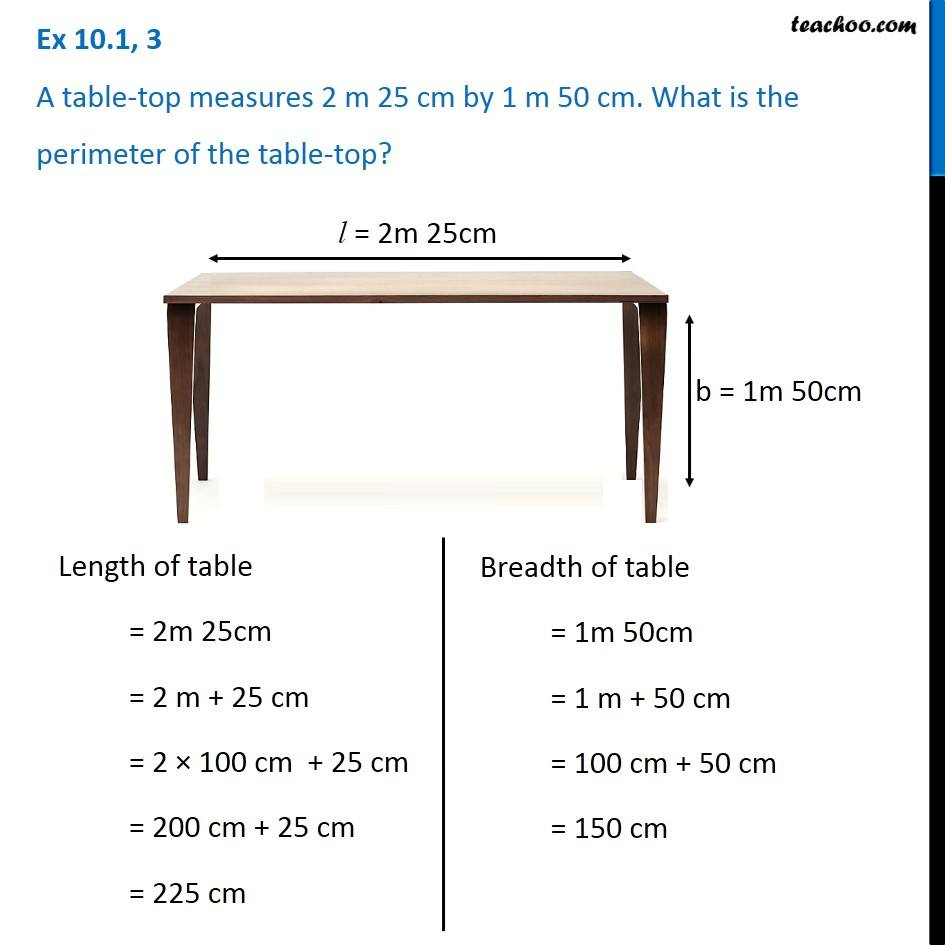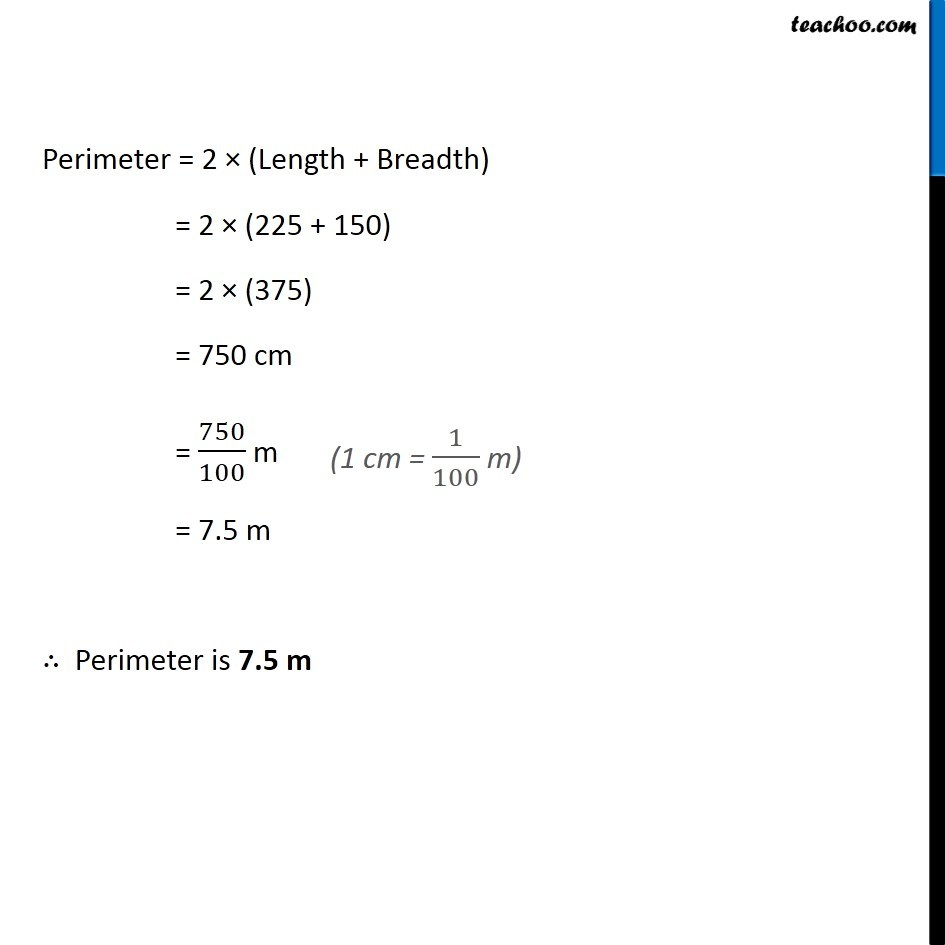Ex 10.1

Chapter 10 Class 6 Mensuration
Serial order wiseGet live Maths 1-on-1 Classs - Class 6 to 12

### Transcript

Ex 10.1, 3 A table-top measures 2 m 25 cm by 1 m 50 cm. What is the perimeter of the table-top? Length of table = 2m 25cm = 2 m + 25 cm = 2 × 100 cm + 25 cm = 200 cm + 25 cm = 225 cm Breadth of table = 1m 50cm = 1 m + 50 cm = 100 cm + 50 cm = 150 cm Perimeter = 2 × (Length + Breadth) = 2 × (225 + 150) = 2 × (375) = 750 cm = 750/100 m = 7.5 m ∴ Perimeter is 7.5 m